Courses

# Binary Search Trees MCQ - 2

## 20 Questions MCQ Test RRB JE for Computer Science Engineering | Binary Search Trees MCQ - 2

Description
This mock test of Binary Search Trees MCQ - 2 for Computer Science Engineering (CSE) helps you for every Computer Science Engineering (CSE) entrance exam. This contains 20 Multiple Choice Questions for Computer Science Engineering (CSE) Binary Search Trees MCQ - 2 (mcq) to study with solutions a complete question bank. The solved questions answers in this Binary Search Trees MCQ - 2 quiz give you a good mix of easy questions and tough questions. Computer Science Engineering (CSE) students definitely take this Binary Search Trees MCQ - 2 exercise for a better result in the exam. You can find other Binary Search Trees MCQ - 2 extra questions, long questions & short questions for Computer Science Engineering (CSE) on EduRev as well by searching above.
QUESTION: 1

### A Binary Search Tree (BST) stores values in the range 37 to 573. Consider the following sequence of keys. I. 81, 537, 102, 439, 285, 376, 305 II. 52, 97, 121, 195, 242, 381, 472 III. 142, 248, 520, 386, 345, 270, 307 IV. 550, 149, 507, 395, 463, 402, 270 Q. Suppose the BST has been unsuccessfully searched for key 273. Which all of the above sequences list nodes in the order in which we could have encountered them in the search?

Solution:

Key to be searched 273:

• I) 81, 537, 102, 439, 285, 376, 305 is not correct
We cannot go to 376 from 285 as 273 is smaller than 285.
• II) 52, 97, 121, 195, 242, 381, 472 is not correct.
We cannot go to 472 from 381 as 273 is smaller than 381.
• III) 142, 248, 520, 386, 345, 270, 307 is correct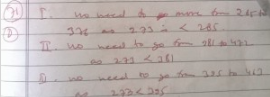• 550, 149, 507, 395, 463, 402, 270 is not correct. We cannot go to 463 from 395 in search of 273
QUESTION: 2

### A Binary Search Tree (BST) stores values in the range 37 to 573. Consider the following sequence of keys.  I. 81, 537, 102, 439, 285, 376, 305  II. 52, 97, 121, 195, 242, 381, 472  III. 142, 248, 520, 386, 345, 270, 307  IV. 550, 149, 507, 395, 463, 402, 270  Q. Which of the following statements is TRUE?

Solution:

A: I and IV are not in ascending order
B: If 439 is root, It should be 1st element in preorder
D: IV is a postorder sequence of some BST with 149 as the root, => False

QUESTION: 3

### How many distinct BSTs can be constructed with 3 distinct keys?

Solution:

2nCn/(n+1) = 6C3/4 = 5

QUESTION: 4

What is the worst case possible height of Red-Black tree? Assume base of Log as 2 in all options

Solution:
QUESTION: 5

Is the following statement valid? A Red-Black Tree which is also a perfect Binary Tree can have all black nodes

Solution:

A perfect BST with all black nodes doesn't violate any of the Red-Black tree properties.

QUESTION: 6

Which of the following operations are used by Red-Black trees to maintain balance during insertion/deletion?
a) Recoloring of nodes
b) Rotation (Left and Right)

Solution:

Both recoloring and rotation operations are used during insertion and deletion.

QUESTION: 7

A program takes as input a balanced binary search tree with n leaf nodes and computes the value of a function g(x) for each node x. If the cost of computing g(x) is min{no. of leaf-nodes in left-subtree of x, no. of leaf-nodes in right-subtree of x} then the worst-case time complexity of the program is

Solution:

The recurrence relation for the recursive function is T(N) = 2 * T(N/2) + n/2
Where N is the total no. of nodes in the tree.
T(N) = 2 * (2*T(N/2) + n/2) + n/2
= 4 * T(N/2) + 3(n/2)
Solve this till T(1) i.e. till we reach the root.
T(N) = c * T(N / 2^i) + (2*i - 1) * (n/2)
Where i = lg(N)
= lg((2n - 1) / 2)
O(c * T(N / 2^i) + (2*i - 1) * (n/2)) reduces to
O((2*i - 1) * (n/2))
O((2*( lg((2n - 1) / 2)) - 1) * (n/2)) ...sub the value of i.
O(n * ln(n))

QUESTION: 8

Which of the following is TRUE?

Solution:

AVL tree is a balanced tree.
AVL tree's time complexity of searching = θ(logn)
But a binary search tree, may be skewed tree, so in worst case BST searching time = θ(n)

QUESTION: 9

Given two Balanced binary search trees, B1 having n elements and B2 having m elements, what is the time complexity of the best known algorithm to merge these trees to form another balanced binary tree containing m+n elements ?

Solution:

O(m+n) as we can first perform inorder on both the trees and store them in two separate arrays. Now we have two sorted sequences and we can merge them in O(m+n) using standard merge algorithm and on the final sorted array we can use the binary search to create the tree using Recursion. Recursively adding middle element at the root and repeating the same process for left and right subarrays.

QUESTION: 10

The worst case running time to search for an element in a balanced in a binary search tree with n2^n elements is

Solution:

Time taken to search an element is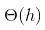where h is the height of Binary Search Tree (BST). The growth of height of a balanced BST is logerthimic in terms of number of nodes. So the worst case time to search an element would be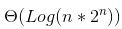which is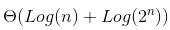Which is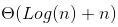] which can be written as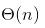.

QUESTION: 11

What is the maximum height of any AVL-tree with 7 nodes? Assume that the height of a tree with a single node is 0.

Solution:

AVL trees are binary trees with the following restrictions. 1) the height difference of the children is at most 1. 2) both children are AVL trees Following is the most unbalanced AVL tree that we can get with 7 nodes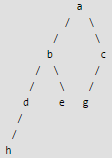QUESTION: 12

What is the worst case possible height of AVL tree?

Solution:
QUESTION: 13

Which of the following is AVL Tree?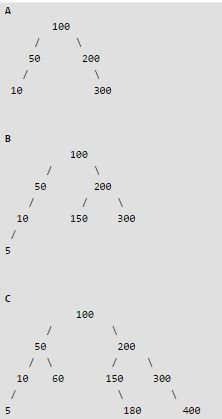Solution:

A Binary Search Tree is AVL if balance factor of every node is either -1 or 0 or 1. Balance factor of a node X is [(height of X->left) - (height of X->right)]. In Tree B, the node with value 50 has balance factor 2. That is why B is not an AVL tree.

QUESTION: 14

Consider the following AVL tree.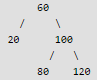Q. Which of the following is updated AVL tree after insertion of 70

Solution:

Refer following for steps used in AVL insertion. AVL Tree | Set 1 (Insertion)

After insertion of 70, tree becomes following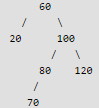We start from 50 and travel up. We keep travelling up till we find an unbalanced node. In above case, we reach the node 60 and see 60 got unbalanced after insertion and this is Right Left Case. So we need to apply two rotations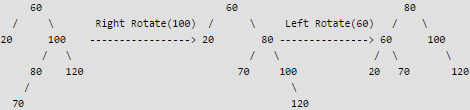QUESTION: 15

Which of the following is a self-adjusting or self-balancing Binary Search Tree

Solution:

See Splay Tree, AVL Tree and Red Black Tree

QUESTION: 16

Consider the following left-rotate and right-rotate functions commonly used in self-adjusting BSTs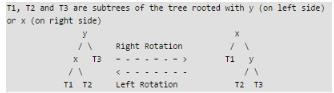Q.
Which of the following is tightest upper bound for left-rotate and right-rotate operations.

Solution:

The rotation operations (left and right rotate) take constant time as only few pointers are being changed there. Following are C implementations of left-rotate and right-rotate 1

QUESTION: 17

Which of the following is true

Solution:

Red Black Tree with n nodes has height <= 2Log2(n+1) AVL Tree with n nodes has height less than Logφ(√5(n+2)) - 2. Therefore, the AVL trees are more balanced compared to Red Black Trees, but they may cause more rotations during insertion and deletion. So if your application involves many frequent insertions and deletions, then Red Black trees should be preferred. And if the insertions and deletions are less frequent and search is more frequent operation, then AVL tree should be preferred over Red Black Tree.

QUESTION: 18

Which of the following is true about Red Black Trees?

Solution:
QUESTION: 19

Which of the following is true about AVL and Red Black Trees?

Solution:

Refer Red Black Tree Insertion and AVL Tree Insertion

QUESTION: 20

The number of edges from the root to the node is called __________ of the tree.

Solution: